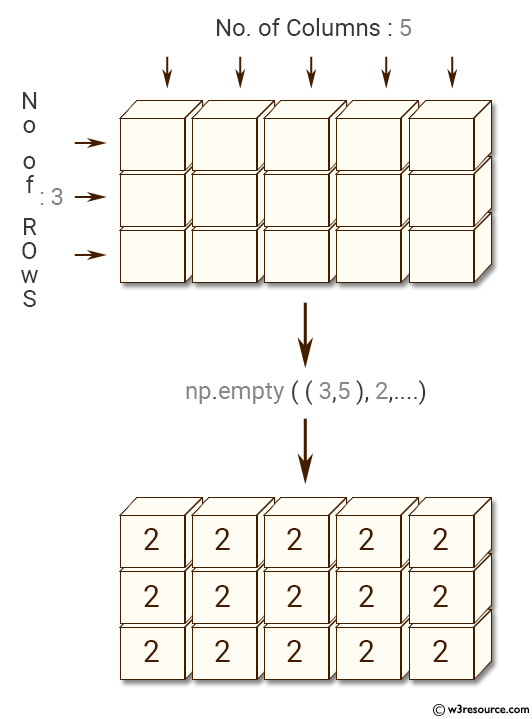﻿ NumPy: Create a new array of 3*5, filled with 2 - w3resource# NumPy: Create a new array of 3*5, filled with 2

## NumPy: Array Object Exercise-40 with Solution

Write a NumPy program to create a new array of 3*5, filled with 2.

Pictorial Presentation:Sample Solution:-

Python Code:

``````import numpy as np
#using no.full
x = np.full((3, 5), 2, dtype=np.uint)
print(x)
#using no.ones
y = np.ones([3, 5], dtype=np.uint) *2
print(y)
```
```

Sample Output:

```[[2 2 2 2 2]
[2 2 2 2 2]
[2 2 2 2 2]]
[[2 2 2 2 2]
[2 2 2 2 2]
[2 2 2 2 2]]
```

Python Code Editor:

Have another way to solve this solution? Contribute your code (and comments) through Disqus.

What is the difficulty level of this exercise?

Test your Python skills with w3resource's quiz

﻿

## Python: Tips of the Day

List comprehension:

```>>> m = [x ** 2 for x in range(5)]
>>> m
[0, 1, 4, 9, 16]
```